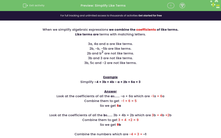# Simplify Like Terms

In this worksheet, students will simplify like algebraic terms involving several variables.Key stage:  KS 3

Curriculum topic:   Algebra

Curriculum subtopic:   Use Standard Mathematical Formulae

Difficulty level:#### Worksheet Overview

When we simplify algebraic expressions, we combine like terms.

Like terms are terms with matching letters.

3a, 4a and a are like terms because they are all multiples of a.

2b, -b, -5b are like terms because they are all multiples of a - even though some of them are negative.

2b and b2 are not like terms: 2b means 2 x b and b2  means b x b.

3b and 3 are not like terms: 3b is a multiple of b and 3 is not.

3b, 5c and -2 are not like terms: there is a multiple of b, a multiple of c and a number.

Example

Simplify -4 + 3b + 4b - a + 2b + 6a + 3

Look at the values before all the as (known as the coefficients):  -a + 6a which are -1a + 6a

Combine them to get  -1 + 6 = 5

So we get 5a

Look at the coefficients of all the bs...... 3b + 4b + 2b which are 3b + 4b + 2b

Combine them to get 3 + 4  + 2 = 9

So we get 9b

Combine the numbers which are -4 + 3 = -1

The answer is 5a + 9b - 1

Let's have a go at some questions.

### What is EdPlace?

We're your National Curriculum aligned online education content provider helping each child succeed in English, maths and science from year 1 to GCSE. With an EdPlace account you’ll be able to track and measure progress, helping each child achieve their best. We build confidence and attainment by personalising each child’s learning at a level that suits them.

Get started